## 【C++初阶】C++基础（一）

news/2023/6/6 5:11:26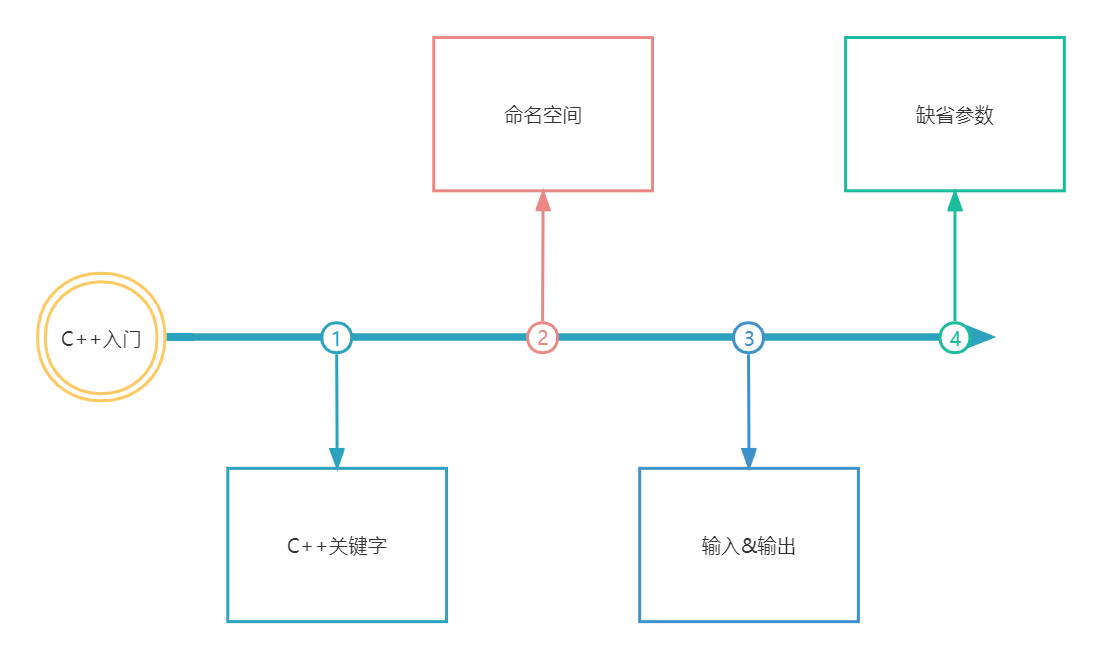C++是在C的基础之上，容纳进去了面向对象编程思想，并增加了许多有用的库，以及编程范式

1. 补充C语言语法的不足，以及C++是如何对C语言设计不合理的地方进行优化的，比如：作用

2. 为后续类和对象学习打基础。

# 1. C++关键字(C++98)

C++总计63个关键字，C语言32个关键字
ps：下面我们只是看一下C++有多少关键字，不对关键字进行具体的讲解。后面我们学到以后再细说。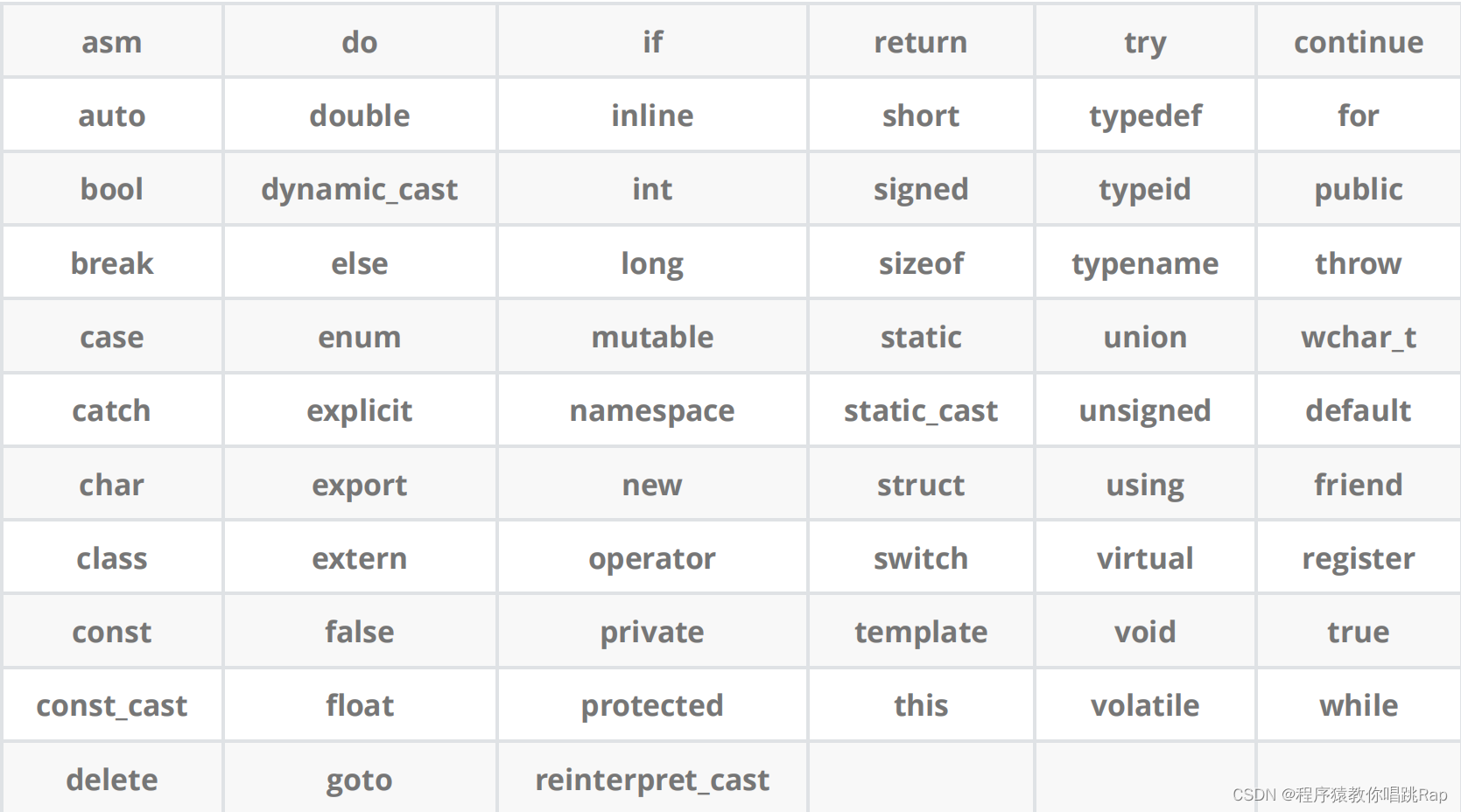# 2. 命名空间

``````#include <stdio.h>
#include <stdlib.h>
int rand = 10;
// C语言没办法解决类似这样的命名冲突问题，所以C++提出了namespace来解决
int main()
{printf("%d\n", rand);return 0;
}
//编译后后报错：error C2365: “rand”: 重定义；以前的定义是“函数”``````

## 2.1 命名空间定义

``````// 1. 正常的命名空间定义
namespace tt
{// 命名空间中可以定义变量/函数/类型int rand = 10;int Add(int left, int right){return left + right;}struct Node{struct Node* next;int val;};
}
//2. 命名空间可以嵌套
// test.cpp
namespace N1
{int a;int b;int Add(int left, int right){return left + right;}namespace N2{int c;int d;int Sub(int left, int right){return left - right;}}
}
//3. 同一个工程中允许存在多个相同名称的命名空间,编译器最后会合成同一个命名空间中。
// ps：一个工程中的test.h和上面test.cpp中两个N1会被合并成一个
// test.h
namespace N1
{int Mul(int left, int right){return left * right;}
}``````

## 2.2 命名空间使用

``````namespace ft
{// 命名空间中可以定义变量/函数/类型int a = 0;int b = 1;int Add(int left, int right){return left + right;}struct Node{struct Node* next;int val;};
}
int main()
{// 编译报错：error C2065: “a”: 未声明的标识符printf("%d\n", a);return 0;
}``````

• 加命名空间名称及作用域限定符

``````int main()
{printf("%d\n", N::a);return 0;
}``````
• 使用using将命名空间中某个成员引入

``````using N::b;
int main()
{printf("%d\n", N::a);printf("%d\n", b);return 0;
}``````
• 使用using namespace 命名空间名称引入

``````using namespce N;
int main()
}``````

# 3. C++输入&输出

C语言中，我们使用scanf和printf来进行输入输出。在C++里面，我们也可以使用scanf和printf来进行输入输出，但是C++本身也有一套输入cin和输出cout
``````#include<iostream>
// std是C++标准库的命名空间名，C++将标准库的定义实现都放到这个命名空间中
using namespace std;
int main()
{cout << "Hello world!!!" << endl;return 0;
}``````

``  // >> 流提取运算符cin>>a;// << 流插入运算符cout<<a;``

``````#include<iostream>
using namespace std;
int main()
{int a;double d;char c;cin >> a >> d >> c;cout << a << ' ' << d << ' ' << c << endl;return 0;
}``````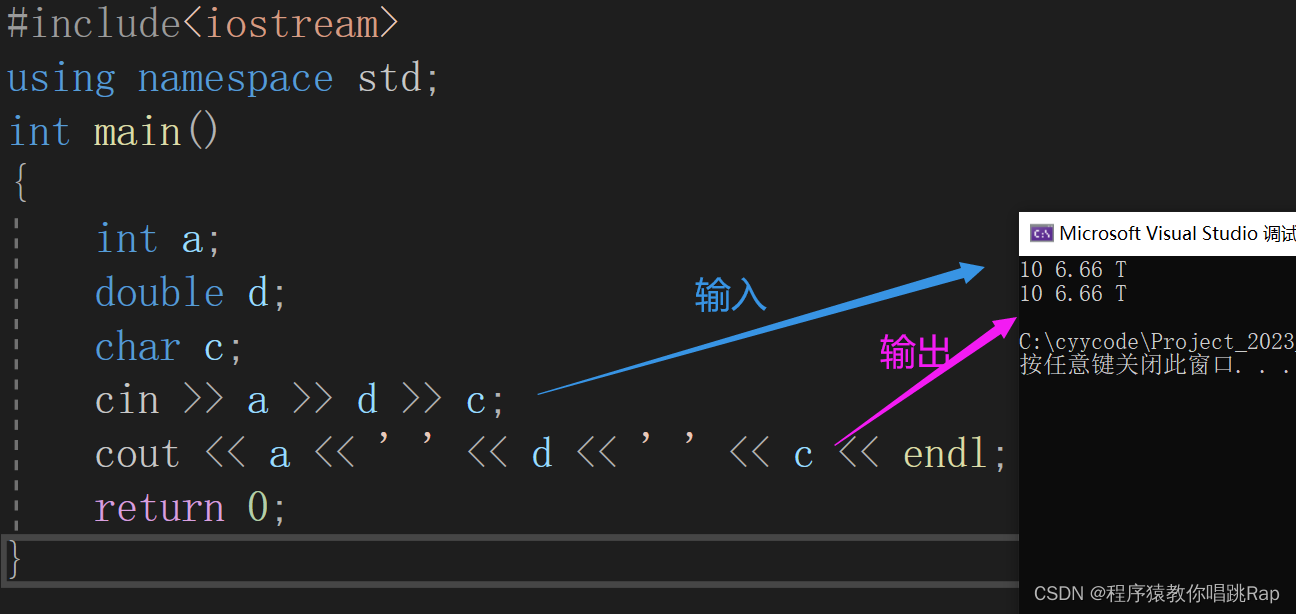【注】这里endl是换行符，等价于C语言里面的'\n'。

# 4.缺省参数

## 4.1 缺省参数概念

``````//缺省参数(默认参数)
void Func(int a = 0)
{cout << a << endl;
}
int main()
{Func();     // 没有传参时，使用参数的默认值Func(10);   // 传参时，使用指定的实参return 0;
}``````

## 4.2 缺省参数分类

1.全缺省
2.半缺省

### 4.2.1 全缺省

``````void Func(int a = 10, int b = 20, int c = 30)
{cout << "a = " << a << endl;cout << "b = " << b << endl;cout << "c = " << c << endl;
}``````

``````int main()
{Func();//全用调用的Func(1);//a调用的，bc用默认的Func(1, 2);//ab调用的，c用默认的Func(1, 2, 3);//全用默认的
}``````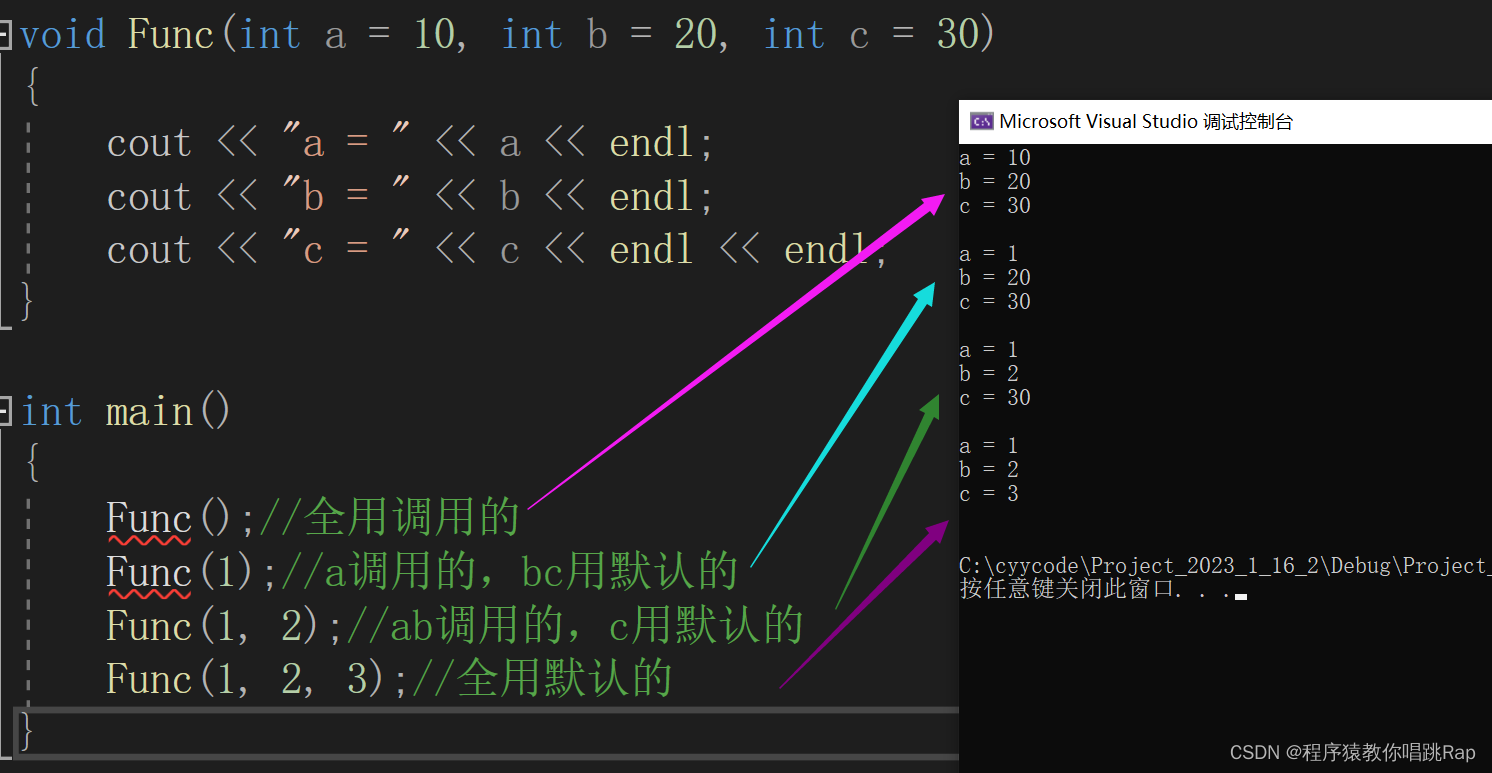【注】传参的时候要按顺序来传
Func( , 2, 3); //error

### 4.2.2 半缺省

``````void Func(int a, int b = 20, int c = 30)
{cout << "a = " << a << endl;cout << "b = " << b << endl;cout << "c = " << c << endl << endl;
}``````

``````int main()
{Func(1);//全用默认的Func(1, 2);//只有c用默认Func(1, 2, 3);//全用自己的
}``````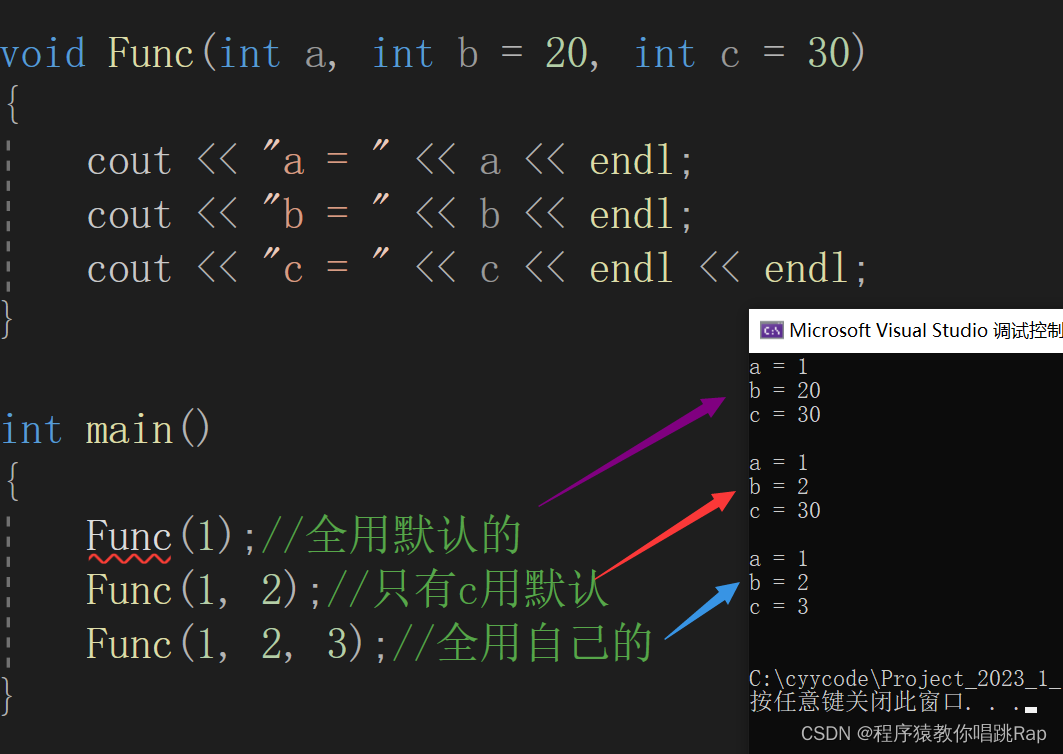【注】
1.半缺省参数必须从右往左依次来给出，不能间隔着给
2.缺省参数不能在函数声明和定义中同时出现
3. 缺省值必须是常量或者全局变量
4. C语言不支持（编译器不支持）

## 4.3 缺省参数的意义

``````//没学C++之前
void stackInit(struct stack* ps)
{//.........
}//通过缺省参数来实现
void stackInit(struct stack* ps, int n = 4)
{assert(ps);ps->a = (int*)malloc(sizeof(int) * n);ps->size = 0;ps->capacity = n;
}``````

``````int main()
{stack st;stackInit(&st);stackInit(&st, 100);return 0;
}``````

### 字节跳动取消大小周后首次发薪，员工炸锅：薪资普降 17%

“有生之年终于等来了字节跳动的普调&#xff0c;全体员工普调降低了17%。” 整理 | 王晓曼 出品 | CSDN&#xff08;ID&#xff1a;CSDNnews&#xff09; 6月底&#xff0c;字节跳动开启取消大小周的内部调查&#xff0c;结果显示1/3员工表示不支持取消大小周&#xff0c;有…

### 快手宣布将实行大小周，打工人何时能正常上下班？

2020年12月30号&#xff0c;快手人力负责人刘峰在快手全员会上宣布&#xff0c;快手将于2021年1月10号全员开启大小周。大小周&#xff0c;即为现今国内部分公司一种适用的休息方式。是指一个星期上六天班&#xff0c;一个星期上五天。刘峰表示&#xff0c;西方周日是一周的开始…